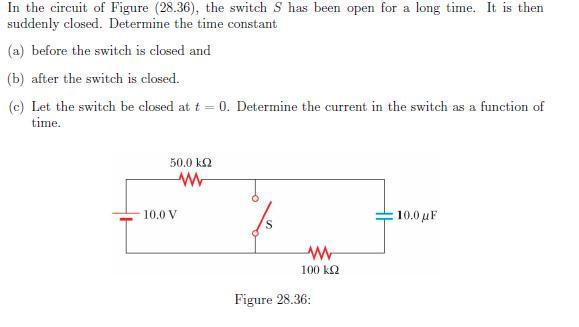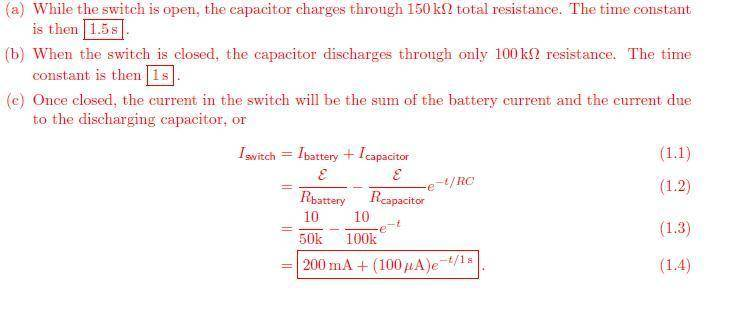# RC circuits - questions about the solution

RC circuits -- questions about the solution

## Homework Statement

First of all , greetings from Peru and thank you to all the people who help another people here,taht's awesome , please help me with this guys... Here's the statementThe solution isMy questions:
ok my question is about the solution for the question "c", it says that the current is the sum of the current produced by the battery and the current produced by the dischargin process,well ,my teacher told me that after that a capacitor is fully charged by a battery , the current is zero because there is no pottential difference between them. so, :

1. Why the solution adds the current produced by the battery? why isn't it zero?Isn't it zero at t=0 too?
2.why they say the current at the closed switch is the sum of both currents whithout drawing directions of both currents?( you know like kirchoff's junction rule,i've checked out some solutions of this problem and they don't draw the directions they just say it's the sum of both currents)

Last edited:

berkeman
Mentor

## Homework Statement

First of all , greetings from Peru and thank you to all the people who help another people here,taht's awesome , please help me with this guys... Here's the statement
View attachment 54129
The solution is View attachment 54130

My questions:
ok my question is about the solution for the question "c", it says that the current is the sum of the current produced by the battery and the current produced by the dischargin process,well ,my teacher told me that after that a capacitor is fully charged by a battery , the current is zero because there is no pottential difference between them. so, :

1. Why the solution adds the current produced by the battery? why isn't it zero?Isn't it zero at t=0 too?
2.why they say the current at the closed switch is the sum of both currents whithout drawing directions of both currents?( you know like kirchoff's junction rule,i've checked out some solutions of this problem and they don't draw the directions they just say it's the sum of both currents)

Before the switch is closed, the cap is charged up so no more current flows (Vcap = Vbat, so there is no voltage difference across the top resistor to drive any current).

After the switch is closed, the battery drives current through the top resistor to ground through the switch (call the bottom of the circuit "ground"), and the capacitor discharges through the bottom right resistor through the switch. That's why for a time there are two currents adding up through the switch. After the cap fully discharges, only the battery supplies any current through the switch.

Make sense?

Before the switch is closed, the cap is charged up so no more current flows (Vcap = Vbat, so there is no voltage difference across the top resistor to drive any current).

After the switch is closed, the battery drives current through the top resistor to ground through the switch (call the bottom of the circuit "ground"), and the capacitor discharges through the bottom right resistor through the switch. That's why for a time there are two currents adding up through the switch. After the cap fully discharges, only the battery supplies any current through the switch.

Make sense?

Thanks for your reply but I still don't get it ,why does the battery still 'drive' current when it's supossed to be zero, ? the solution says that the capacitor charges through both resistances , so after the capacitor is charged up and the switch is closed , the current at the circuit should be still zero ,right ? i'm confusedit would be great if you tell me why they didn't draw the directions of both currents too. thank you very much .

Last edited:
berkeman
Mentor

After the switch is closed at t=0+, you basically have two seperate circuits. Because of the short circuit in the middle, no voltage information is transferred across a short circuit.

So redraw the circuit for t=0+. Make it two separate circuits. The left circuit is a power supply driving a constant voltage across a constant resistance, so that makes a constant current. V=IR.

And the right side circuit is a charged up capacitor in series with a resistor. So you get an exponentially decreasing voltage as the cap discharges, so you get an exponentially decreasing current through the resistor versus time. V=IR.

After the switch is closed at t=0+, you basically have two seperate circuits. Because of the short circuit in the middle, no voltage information is transferred across a short circuit.

So redraw the circuit for t=0+. Make it two separate circuits. The left circuit is a power supply driving a constant voltage across a constant resistance, so that makes a constant current. V=IR.

And the right side circuit is a charged up capacitor in series with a resistor. So you get an exponentially decreasing voltage as the cap discharges, so you get an exponentially decreasing current through the resistor versus time. V=IR.

Thanks , so.. since at t=0 is like we have two separate circuits then the current at the switch will be like the sum of the current of every circuit ,right? why directions is not important here?

why directions is not important here?

it is important. both currents add because they flow in the same direction.

ehild
Homework Helper

Thanks , so.. since at t=0 is like we have two separate circuits then the current at the switch will be like the sum of the current of every circuit ,right? why directions is not important here?

The direction is important, that is, why the currents add.

The upper terminal of the battery is positive. The current of the battery flows through the 50 kΩ resistor and the switch towards the negative terminal. That means a downward current through the switch.

The capacitor is charged, and its upper plate is positive. When the switch is closed, the charge flows away from it, the excess positive charge flows from the positive plate through the switch and 100 kΩ resistor towards the negative plate.
That current also flows downward through the switch.

ehild

Thank you guys . That was a great explanation .thanks